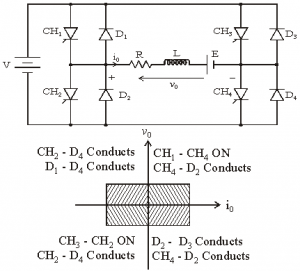Home > dc dc converter chopper > classification of chopper

# Classification of Chopper

Depending upon the direction of the output current and voltage, the converters can be classified into five classes namely
â€¢ Class D Chopper [Two-quadrant Operation]
â€¢ Class E Chopper [Four-quadrant Operation]

â€¢ Class A Chopper is a first quadrant chopper
â€¢ When chopper is ON, supply voltage V is connected across the load.
â€¢ When chopper is OFF, vO = 0 and the load current continues to flow in the same direction through the FWD.
â€¢ The average values of output voltage and current are always positive. Class A Chopper is a first quadrant chopper
â€¢ When chopper is ON, supply voltage V is connected across the load.
â€¢ When chopper is OFF, vO = 0 and the load current continues to flow in the same direction through the FWD.
â€¢ The average values of output voltage and current are always positive.
â€¢ Class A Chopper is a step-down chopper in which power always flows form source to load.
â€¢ It is used to control the speed of dc motor.
â€¢ The output current equations obtained in step down chopper with R-L load can be used to study the performance of Class A Chopper.

Figure below shoesÂ  Class A chopper.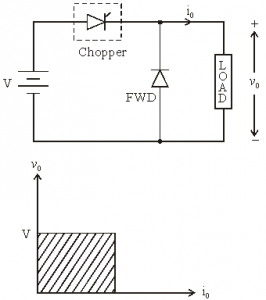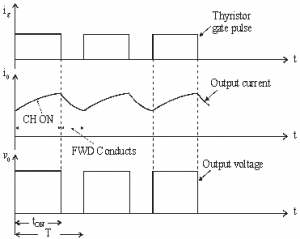â€¢ Class B Chopper is a step-up chopper
â€¢ When chopper is ON, E drives a current through L and R in a direction opposite to that shown in figure.
â€¢ During the ON period of the chopper, the inductance L stores energy.
â€¢ When Chopper is OFF, diode D conducts, and part of the energy stored in inductor L is returned to the supply.
â€¢ Average output voltage is positive. Average output current is negative.
â€¢ Therefore Class B Chopper operates in second quadrant.
â€¢ In this chopper, power flows from load to source.
â€¢ Class B Chopper is used for regenerative braking of dc motor.

Figure below shoesÂ  Class B chopper.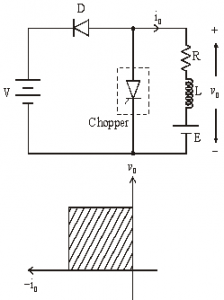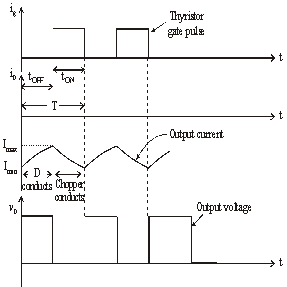â€¢ Class C Chopper can be used as a step-up or step-down chopper
â€¢ Class C Chopper is a combination of Class A and Class B Choppers.
â€¢ For first quadrant operation, CH1 is ON or D2 conducts.
â€¢ For second quadrant operation, CH2 is ON or D1 conducts.
â€¢ When CH1 is ON, the load current is positive.
â€¢ The output voltage is equal to â€˜Vâ€™ & the load receives power from the source.
â€¢ When CH1 is turned OFF, energy stored in inductance L forces current to flow through the diode D2 and the output voltage is zero.
â€¢ Current continues to flow in positive direction.
â€¢ When CH2 is triggered, the voltage E forces current to flow in opposite direction through L and CH2 .
â€¢ The output voltage is zero.
â€¢ On turning OFF CH2 , the energy stored in the inductance drives current through diode D1 and the supply
â€¢ Output voltage is V, the input current becomes negative and power flows from load to source.
â€¢ Average output voltage is positive
â€¢ Average output current can take both positive and negative values.
â€¢ Choppers CH1 & CH2 should not be turned ON simultaneously as it would result in short circuiting the supply.
â€¢ Class C Chopper can be used both for dc motor control and regenerative braking of dc motor.

Figure below shoesÂ  Class C chopper.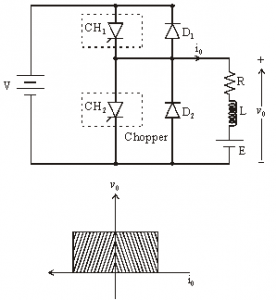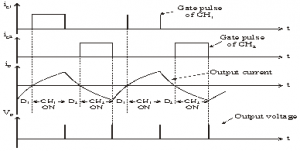â€¢ Class D is a two quadrant chopper.
â€¢ When both CH1 and CH2 are triggered simultaneously, the output voltage vO = V and output current flows through the load.
â€¢ When CH1 and CH2 are turned OFF, the load current continues to flow in the same direction through load, D1 and D2 , due to the energy stored in the inductor L.
â€¢ Output voltage vO = – V.
â€¢ Average load voltage is positive if chopper ON time is more than the OFF time
â€¢ Average output voltage becomes negative if tON < tOFF .
â€¢ Hence the direction of load current is always positive but load voltage can be positive or negative.

Figure below shoesÂ  Class D chopper.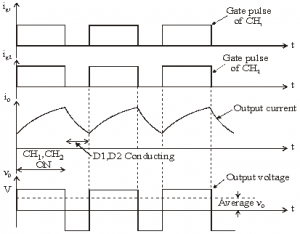â€¢ Class E is a four quadrant chopper
â€¢ When CH1 and CH4 are triggered, output current iO flows in positive direction through CH1 and CH4, and with output voltage vO = V.
â€¢ This gives the first quadrant operation.
â€¢ When both CH1 and CH4 are OFF, the energy stored in the inductor L drives iO through D2 and D3 in the same direction, but output voltage vO = -V.
â€¢ Therefore the chopper operates in the fourth quadrant.
â€¢ When CH2 and CH3 are triggered, the load current iO flows in opposite direction & output voltage vO = -V.
â€¢ Since both iO and vO are negative, the chopper operates in third quadrant.
â€¢ When both CH2 and CH3 are OFF, the load current iO continues to flow in the same direction D1 and D4 and the output voltage vO = V.
â€¢ Therefore the chopper operates in second quadrant as vO is positive but iO is negative.

Figure below shoesÂ  Class E chopper.